Engineering ToolBox - Resources, Tools and Basic Information for Engineering and Design of Technical Applications!

# Discrete Compounding Cash Flow Formulas

## Discrete payments compounding equations and online calculators .

### Single Payment

#### Compound Amount

Converts a single payment (or value) today - to a future value.

F = P [(1 + i)n]                  (1)

where

F = future value

P = single payment today

i = interest rate per period

n = number of periods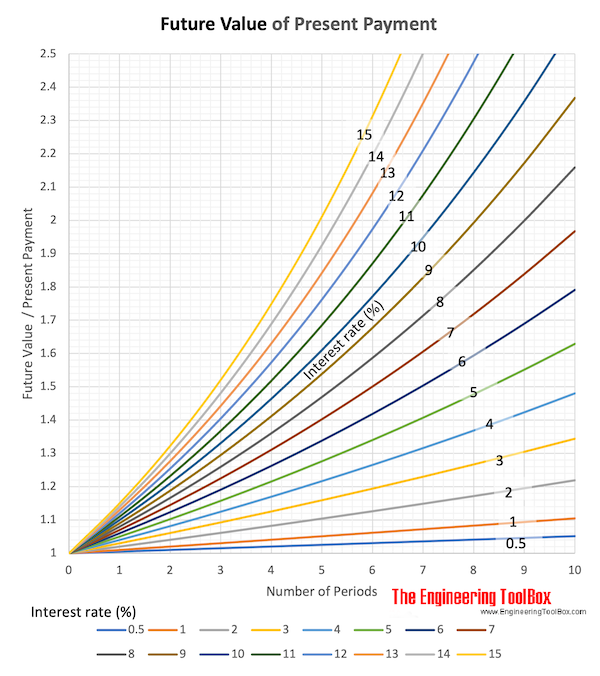##### Example - Future Value of an Initial Amount Received Today

An amount of 5000 is received today. Calculate the future value of this amount after 7 years with interest rate 5%.

The interest rate can be calculated

i = (5 %) / /100 %)

= 0.05

The future value of the amount can be calculated

F = (5000) [(1 + 0.05)7]

= 7036

##### Future Value - Online Calculator

P - single payment today

i - interest (%)

n - number of periods

Note that interest rate in % is used in the calculator - not in the equation.

#### Present Worth (or Value)

Converts a future payment (or value) - to present wort (or value).

P = F [(1 + i)-n]                  (2)

where

P = present value

F = single future payment

i = discount rate per period

n = number of periods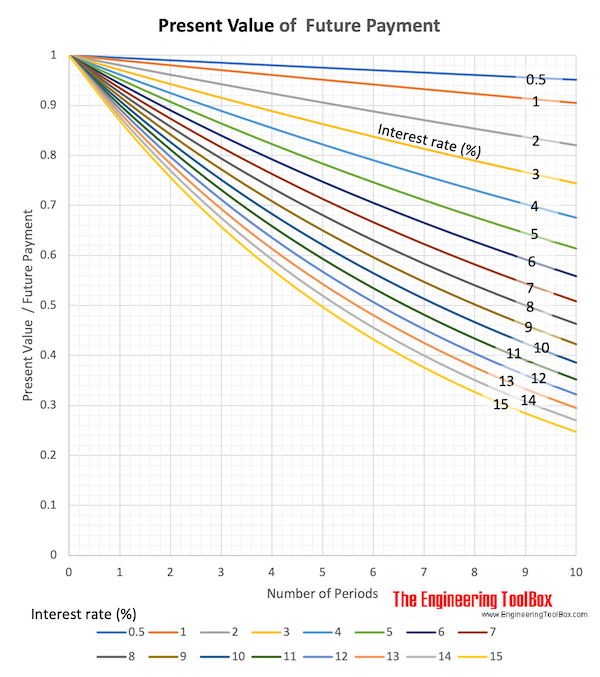##### Example - Present Value of a Future Payment

An payment of 5000 is received after 7 years. Calculate the present worth (or value) of this payment with dicount rate 5%.

The discount rate can be calculated

i = (5 %) / /100 %)

= 0.05

The present worth of the future payment can be calculated

F = (5000) [(1 + 0.05)-7]

= 3553

##### Present Value - Online Calculator

F - single future payment

i - discount rate (%)

n - number of periods

Note that discount rate in % is used in the calculator - not in the equation.

### Uniform Series

#### Compound Amount - Annuity

Converts a uniform amount (annuity) - to a future value.

F = A [((1 + i)n - 1) / i ]                 (3)

where

F = future value

A = uniform amount per period

i = interest rate

n = numbers of periods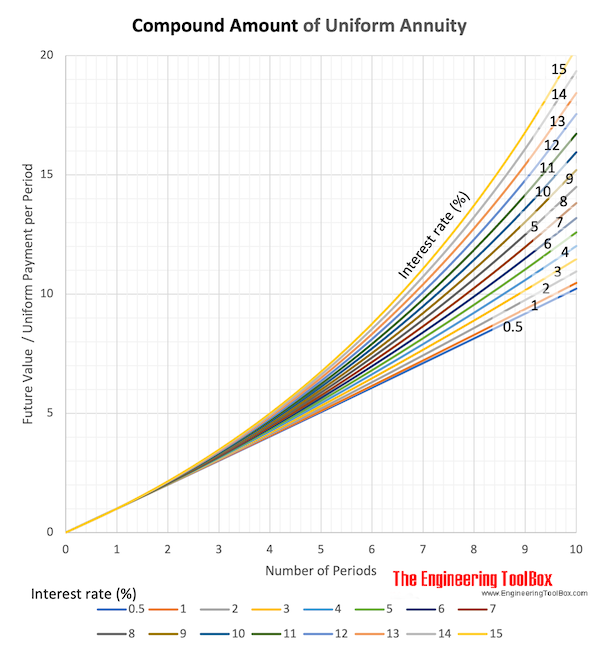Download and print Compound Ammount of Uniform Annuity chart

##### Example - Present Value of Uniforms Payments

An uniform amount of 5000 is paid every year in 7 years. Calculate the future value of this amount with interest rate 5%.

The interest rate can be calculated

i = (5 %) / /100 %)

= 0.05

The future value of the annuity can be calculated

F = 5000 [((1 + 0.05)7 - 1 ) / 0.05]

= 40710

##### Compound Amount - Online Calculator

A - uniform amount per period

i - interest rate (%)

n - number of periods

Note that interest rate ín % is used in the calculator - not in the equation.

#### Sinking Fund

Converts a specific future value to uniform amounts (annuities).

A = F [i / ((1 + i)n - 1)]                 (4)

where

A = uniform amount per period

F = future value

i = interest rate

n = number of periods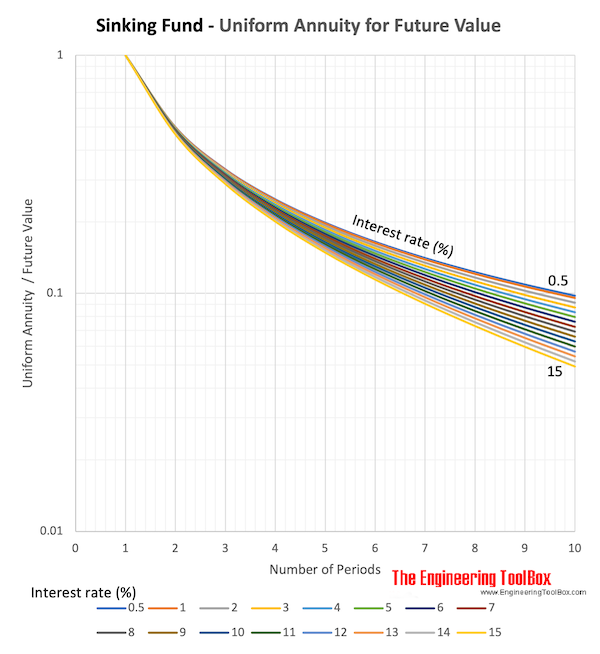Download and print Sinking Fund - Uniform Annuity to Future Value chart

##### Example - Uniforms Payments required to reach a Future Value

The future value of a 7 years annuity is 5000. Calculate the required annuity to reach this value with interest rate 5%.

The interest rate can be calculated

i = (5 %) / /100 %)

= 0.05

The uniform payments (annuity) can be calculated

A = 5000 [0.05 / ((1 + 0.05)7 - 1)]

= 614

##### Sinking Fund - Online Calculator

F - future value

i - interest rate (%)

n - number of periods

Note that interest rate in % is used in the calculator - not in the equation.

#### Present Worth

Converts a uniform amount (annuity) - to a present value.

P = A [((1 + i)n - 1) / ( i (1  + i)n )]                   (5)

where

P = present value

A = amount per interest period

i = discount rate

n = discount periods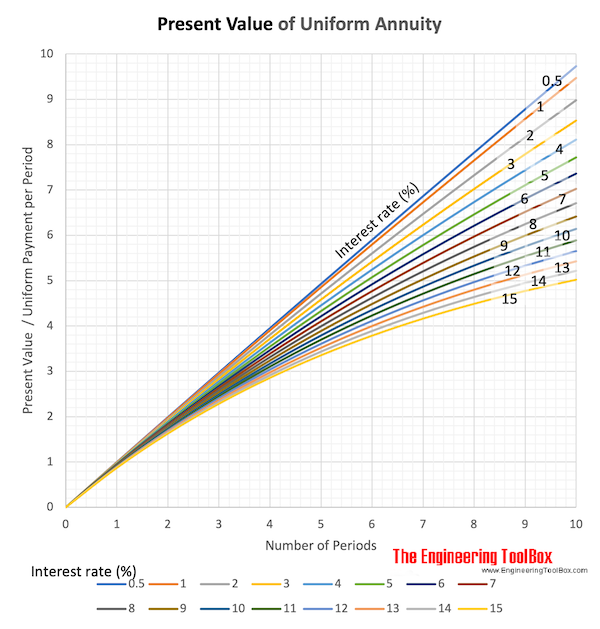##### Example - Present Value of Uniform Amounts

The uniform amount (annuity) paid from a 7 years project is 5000. Calculate the present value with interest rate 5%.

The interest rate can be calculated

i = (5 %) / /100 %)

= 0.05

The present value of the uniform amounts can be calculated

P = 5000 [((1 + 0.05)7 - 1) / ( 0.05 (1 + 0.05)7 )]

= 28932

##### Present Worth or Value - Online Calculator

A - uniform amount per interest period

i - discount rate (%)

n - number of periods

Note that discount rate % is used in the calculator - not in the equation.

#### Capital Recovery

Converts a present value - to a uniform amount (annuity).

A = P [(i (1 + i)n) / ((1+i)n - 1)]                (6)

where

P = present value

A = amount per interest period

i = interest rate

n = discount periods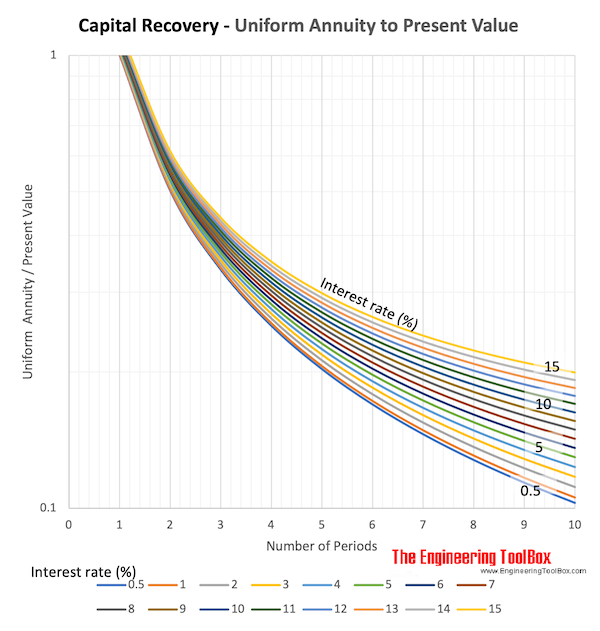Download and print Capital Recovery - Uniform Annuity to Present Value

##### Capital Recovery - Online Calculator

P - present value

i - interest rate (%)

n - number of periods

Note that interest rate in % is used in the calculator - not in the equation.

## Related Topics

• Economics - Engineering economics - cash flow diagrams, present value, discount rates, internal rates of return - IRR, income taxes, inflation.

## Engineering ToolBox - SketchUp Extension - Online 3D modeling!

Add standard and customized parametric components - like flange beams, lumbers, piping, stairs and more - to your Sketchup model with the Engineering ToolBox - SketchUp Extension - enabled for use with the amazing, fun and free SketchUp Make and SketchUp Pro .Add the Engineering ToolBox extension to your SketchUp from the SketchUp Pro Sketchup Extension Warehouse!

Translate

## Privacy

We don't collect information from our users. Only emails and answers are saved in our archive. Cookies are only used in the browser to improve user experience.

Some of our calculators and applications let you save application data to your local computer. These applications will - due to browser restrictions - send data between your browser and our server. We don't save this data.

## Citation

• Engineering ToolBox, (2008). Discrete Compounding Cash Flow Formulas. [online] Available at: https://www.engineeringtoolbox.com/discrete-payments-compounding-formulas-d_1234.html [Accessed Day Mo. Year].

Modify access date.

. .

#### Scientific Online Calculator3 10